Question

# FULL SCREEN PRINTER VERSION ·BACK Chapter 21, Problem 024 Your answer is partially correct. Try again....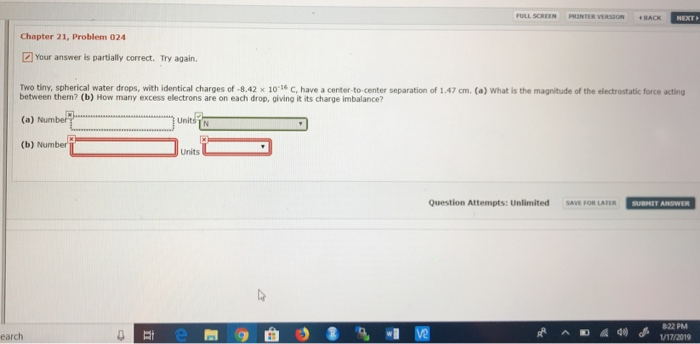FULL SCREEN PRINTER VERSION ·BACK Chapter 21, Problem 024 Your answer is partially correct. Try again. Two tiny, spherical water drops, with identical charges of -8.42 x 10 16 C, have a center-to-center separation of 1.47 cm. (a) What is the magnitude of the electrostatic force acting between them? (b) How many excess electrons are on each drop, giving it its charge imbalance? (b) Number Units Question Attempts: Unlimited SAVE FOR LATER SUBMET ANSWER earch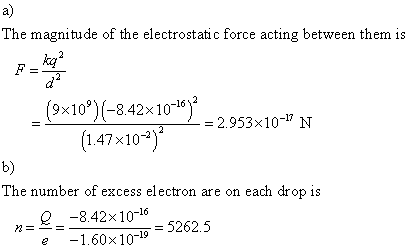#### Earn Coins

Coins can be redeemed for fabulous gifts.

Similar Homework Help Questions
• ### Chapter 21, Problem 024 Two tiny, spherical water drops, with identical charges of-5.71 × 10-16 C,...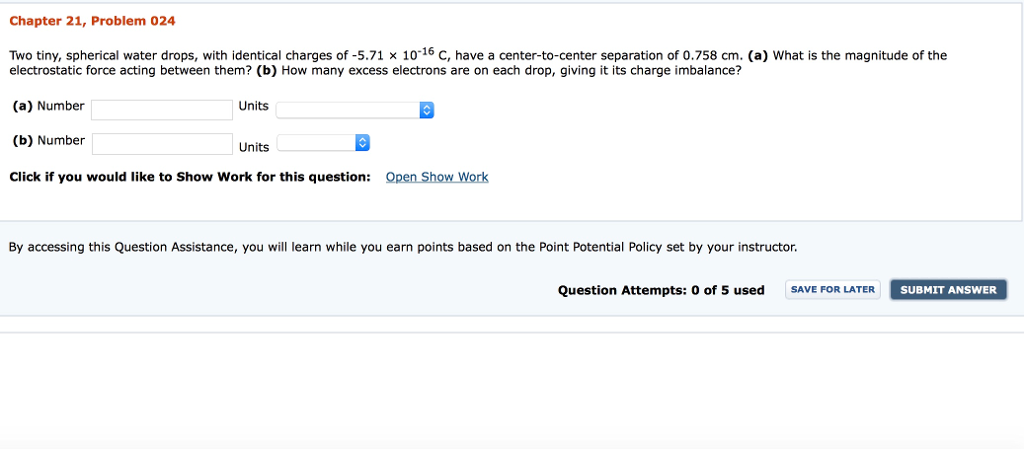Chapter 21, Problem 024 Two tiny, spherical water drops, with identical charges of-5.71 × 10-16 C, have a center-to-center separation of 0.758 cm. (a) what is the magnitude of the electrostatic force acting between them? (b) How many excess electrons are on each drop, giving it its charge imbalance? (a) Number (b) Number Click if you would like to Show Work for this question: Open Show Work Units Units By accessing this Question Assistance, you will learn while you earn...

• ### SCREEN PRINTER VERSION BACK Chapter 21, Problem 042 Your answer is partially correct. Try again. In...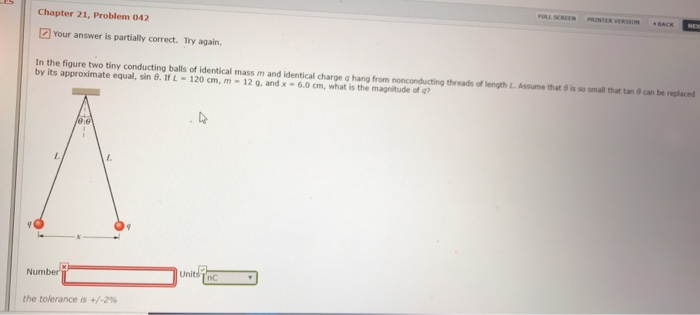SCREEN PRINTER VERSION BACK Chapter 21, Problem 042 Your answer is partially correct. Try again. In the figure two tiny conducting balls of identical mass m and identical charge a hang from no conducting threads of 1 by its approximate equal, sin θ. tfL-120 cm, m-12 g, and x-6.0cm, what h the magnitude of gth L Ass me that。S so sm a at ta e can be replaced Number nC : the tolerance is +/-2%

• ### FUL LSCREEN PRINTER VERSION ACK Chapter 21, Problem 027 The magnltude of the electrostatic frce betvren...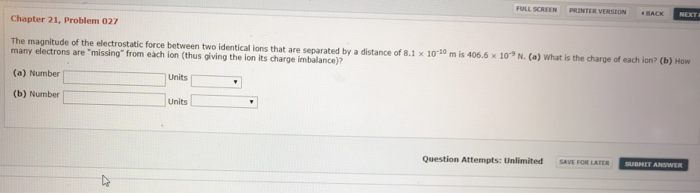FUL LSCREEN PRINTER VERSION ACK Chapter 21, Problem 027 The magnltude of the electrostatic frce betvren two identical ions that are separated by a distance of 8.1 x 1010 m is 406.6x 10" N. Co) What is the charge of eadh ion? (b) How many electrons are "missing" from each ion (thus giving the ion its charge imbalance)? (a) Number (b) Number Units Units Question Attempts: unlimited Dro コ SAVE FOR LATER SUBMET ANSWER

• ### Chapter 21, Problem 069 Your answer is partially correct. Try again. In the radioactive decay (see...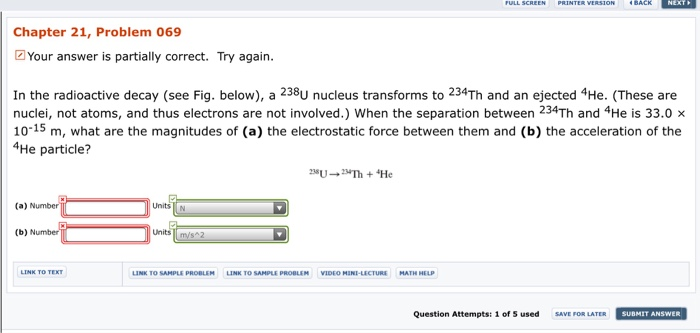Chapter 21, Problem 069 Your answer is partially correct. Try again. In the radioactive decay (see Fig. below), a 238U nucleus transforms to 234Th and an ejected 4He. (These are nuclei, not atoms, and thus electrons are not involved.) When the separation between 234Th and 4He is 33.0>x 10-15 m, what are the magnitudes of (a) the electrostatic force between them and (b) the acceleration of the He particle? (a) Number Units N (b) Number Units Question Attempts: 1 of...

• ### FULL SCREEN PRINTER VERSION BACK Chapter 23, Problem 052 Your answer is partially correct. Try again....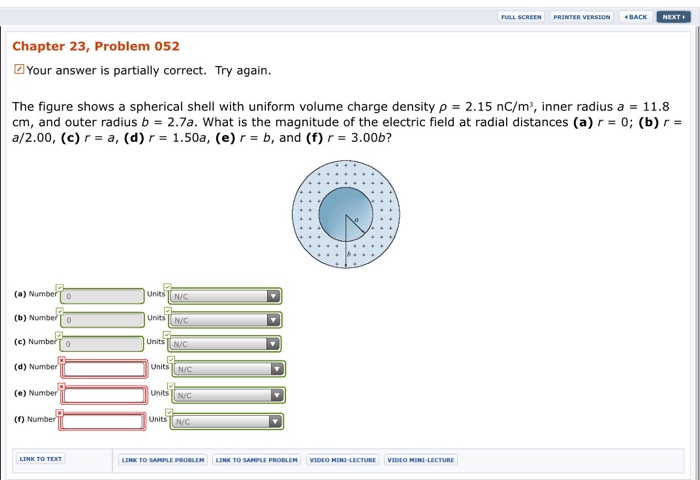FULL SCREEN PRINTER VERSION BACK Chapter 23, Problem 052 Your answer is partially correct. Try again. The figure shows a spherical shell with uniform volume charge density ρ = 2.15 nC/m, inner radius a = 11.8 cm, and outer radius b = 2.7a. what is the magnitude of the electric field at radial distances (a) r = 0; (b) r- a/2.00, (c) r- a, (d) 1.50a, (e)r-b, and (f) r 3.00b? (a) N Units N/C (c) NumberTO (d) Number (e)...

• ### FULL SCREEN PRINTER VERSION < BACK NEXT Chapter 22, Problem 27 Your answer is partially correct....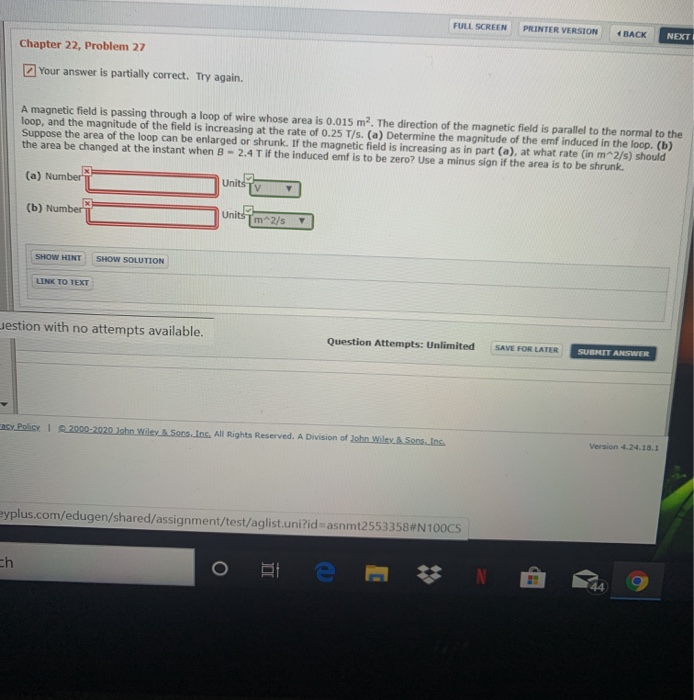FULL SCREEN PRINTER VERSION < BACK NEXT Chapter 22, Problem 27 Your answer is partially correct. Try again. A magnetic field is passing through a loop of wire whose area is 0.015 m2. The direction of the magnetic field is parallel to the normal to the loop, and the magnitude of the field is increasing at the rate of 0.25 T/s. (a) Determine the magnitude of the emf induced in the loop. (b) Suppose the area of the loop can...

• ### wo tiny, spherical water drops, with identical charges of −3.20 ✕ 10−17 C, have a center-to-center...

wo tiny, spherical water drops, with identical charges of −3.20 ✕ 10−17 C, have a center-to-center separation of 1.10 cm. (a) What is the magnitude of the electrostatic force acting between them? ___________________N (b) How many excess electrons are on each drop, giving it its charge imbalance? __________________ electrons

• ### Two tiny, spherical water drops, with identical charges of -5.84 × 10-16 C, have a center-to-center...

Two tiny, spherical water drops, with identical charges of -5.84 × 10-16 C, have a center-to-center separation of 1.12 cm. (a) What is the magnitude of the electrostatic force acting between them? (b) How many excess electrons are on each drop, giving it its charge imbalance?

• ### Two tiny, spherical water drops, with identical charges of -1.17 × 10-16 C, have a center-to-center...

Two tiny, spherical water drops, with identical charges of -1.17 × 10-16 C, have a center-to-center separation of 1.32 cm. (a) What is the magnitude of the electrostatic force acting between them? (b) How many excess electrons are on each drop, giving it its charge imbalance?

• ### Two tiny, spherical water drops, with identical charges of -8.73 × 10-16 C, have a center-to-center...

Two tiny, spherical water drops, with identical charges of -8.73 × 10-16 C, have a center-to-center separation of 1.35 cm. (a) What is the magnitude of the electrostatic force acting between them? (b) How many excess electrons are on each drop, giving it its charge imbalance?